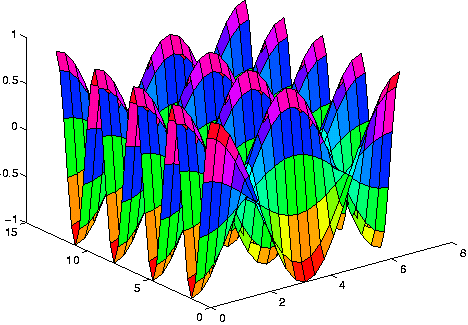# MATLAB

Also found in: Acronyms, Wikipedia.

## MATLAB

(mathematics, language, application)
A high-level language and interactive program from The MathWorks for numeric computation and visualisation. MATLAB supports numerical analysis, matrix computation, signal processing, linear algebra, statistics, Fourier analysis, filtering, optimisation and numerical integration. It can output two and three dimensional graphics and can be integrated with C, C++, Fortran, Java, COM and Microsoft Excel.

http://mathworks.com/products/matlab/.

Latest version: 7.0.4, as of 2005-08-13.

## MATLAB

(MATrix LABoratory) A programming language for technical computing from The MathWorks, Natick, MA (www.mathworks.com). Used for a wide variety of scientific and engineering calculations, especially for automatic control and signal processing, MATLAB runs on Windows, Mac and a variety of Unix-based systems. Developed by Cleve Moler in the late 1970s and based on the original LINPACK and EISPACK FORTRAN libraries, it was initially used for factoring matrices and solving linear equations. Moler commercialized the product with two colleagues in 1984. MATLAB is also noted for its extensive graphics capabilities. The following MATLAB commands generate the 3D graph below:

```     x=(0:2*pi/20:2*pi)';
y=(0:4*pi/40:4*pi)';
[X,Y] = meshgrid(x,y);
z= cos(X).*cos(2*Y);
surf (x,y,z);
```

The Resulting GraphThis graph was computed from the lines of code above and is an example that might be used in multivariate calculus. (Image courtesy of Dr. Mark S. Gockenbach, Michigan Technological University, www.math.mtu.edu/~msgocken/intro/intro.html)
Mentioned in ?
References in periodicals archive ?
MATLAB is a key data analysis tool for many researchers in the Sweden community.
According to MathWorks this new product removes the need to manually translate MATLAB algorithm code into C and C++ code for prototyping, implementation and software integration, resulting in a faster, more efficient system-development workflow.
The NAG toolbox for MATLAB includes more than a dozen MATLAB-based examples of advanced programming for optimisation problems, simulations, time series analyses and other functions important to financial engineering.
Availability for MATLAB for both Microsoft Windows[R] 32-bit & 64-bit and Linux 32-bit & 64-bit; compatability with MATLAB versions 2007a, 2007b and 2008a.
MATLAB users benefit from using the NAG Toolbox for MATLAB by:
MATLAB is a high level language and simulation environment for technical computing.
New data statistics and basic fitting tools allow users to superimpose statistical calculations and curve fits--both text and graphics--directly onto their MATLAB plots.

Site: Follow: Share:
Open / Close